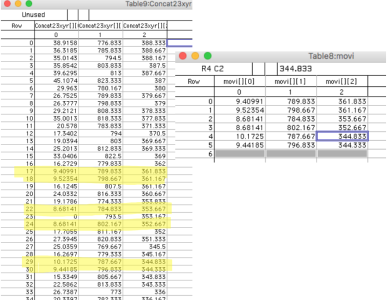# Extracting specific data points from a matrixHello Forum,

I have a matrix (table9) and I want to extract specific data points such that I also copy values in nearby columns (to table8),

for example, in image attached you can see that data from table9 column 0 are picked and corresponding values from column 1 and 2 are also copied.

I can do it for a 1D wave, picking values in column between 7 and 12

Extract /o SourceWave , DestWave , SourceWave >=7 && SourceWave <=12

Now I have a matrix, I want to get the nearby data as well,There might be a faster way, but this one will work:

// make some data
make/N=(20,3) w
w[] = 6 + enoise(6)
w[] = 750 + enoise(50)
w[] = 350 + enoise(20)

MatrixOP/O index = col(w,0)
index = index[p] > 7 && index[p] < 12 ? p : NaN
Wavetransform zapNaNs index
Make/O/N=(numpnts(index),3) output = w[index[p]][q]

And a different way to do, haven't tested speed:

Function extractRows(Wave matrix2d, String newwname, Variable upper, Variable lower)
Duplicate/FREE/R=[] matrix2d, c0
Extract/INDX/FREE c0, indx, (c0 > lower) && (c0 < upper)
Make/N=(numpnts(indx), DimSize(matrix2d,1))/O \$newwname/WAVE=w
w = matrix2d[indx[p]][q]
end